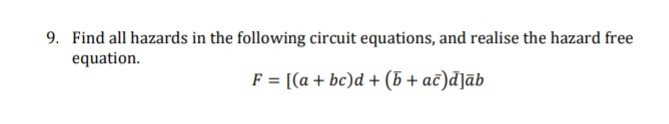# 9. Find all hazards in the following circuit equations, and realise the hazard free equation. F = [(a + bc)d + (5 + ac)d]āb

Questionhelp_outlineImage Transcriptionclose9. Find all hazards in the following circuit equations, and realise the hazard free equation. F = [(a + bc)d + (5 + ac)d]āb fullscreen